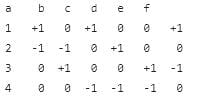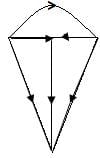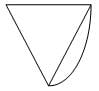Test: Tree and Co-Tree

# Test: Tree and Co-Tree

Test Description

## 10 Questions MCQ Test Network Theory (Electric Circuits) | Test: Tree and Co-Tree

Test: Tree and Co-Tree for Electrical Engineering (EE) 2023 is part of Network Theory (Electric Circuits) preparation. The Test: Tree and Co-Tree questions and answers have been prepared according to the Electrical Engineering (EE) exam syllabus.The Test: Tree and Co-Tree MCQs are made for Electrical Engineering (EE) 2023 Exam. Find important definitions, questions, notes, meanings, examples, exercises, MCQs and online tests for Test: Tree and Co-Tree below.
Solutions of Test: Tree and Co-Tree questions in English are available as part of our Network Theory (Electric Circuits) for Electrical Engineering (EE) & Test: Tree and Co-Tree solutions in Hindi for Network Theory (Electric Circuits) course. Download more important topics, notes, lectures and mock test series for Electrical Engineering (EE) Exam by signing up for free. Attempt Test: Tree and Co-Tree | 10 questions in 30 minutes | Mock test for Electrical Engineering (EE) preparation | Free important questions MCQ to study Network Theory (Electric Circuits) for Electrical Engineering (EE) Exam | Download free PDF with solutions
 1 Crore+ students have signed up on EduRev. Have you?
Test: Tree and Co-Tree - Question 1

### A graph is said to be a directed graph if ________ of the graph has direction.

Detailed Solution for Test: Tree and Co-Tree - Question 1

If every branch of the graph has direction, then the graph is said to be a directed graph. If the graph does not have any direction then that graph is called undirected graph.

Test: Tree and Co-Tree - Question 2

### If no two branches of the graph cross each other, then the graph is called?

Detailed Solution for Test: Tree and Co-Tree - Question 2

If a graph can be drawn on a plane surface such that no two branches of the graph cross each other, then the graph is called planar graph.

Test: Tree and Co-Tree - Question 3

### Number of twigs in a tree are? (where, n-number of nodes)

Detailed Solution for Test: Tree and Co-Tree - Question 3

Twig is a branch in a tree. Number of twigs in a tree are n - 1. If there are 4 nodes in a tree then number of possible twigs are 3.

Test: Tree and Co-Tree - Question 4

If the incident matrix of a graph is given below. The corresponding graph is?Detailed Solution for Test: Tree and Co-Tree - Question 4

For the given incidence matrix,the corresponding graph isconsidering the directions specified in the graph.

Test: Tree and Co-Tree - Question 5

If a graph consists of 5 nodes, then the number of twigs in the tree is?

Detailed Solution for Test: Tree and Co-Tree - Question 5

Number of twigs = n-1. As given number of nodes are 5 then n = 5. On substituting in the equation, number of twigs = 5 -1 = 4.

Test: Tree and Co-Tree - Question 6

If there are 4 branches, 3 nodes then number of links in a co-tree are?

Detailed Solution for Test: Tree and Co-Tree - Question 6

Number of links = b - n + 1. Given number of branches = 4 and number of nodes = 3. On substituting in the equation, number of links in a co-tree = 4 – 3 + 1 = 2.

Test: Tree and Co-Tree - Question 7

If A represents incidence matrix, I represents branch current vectors, then?

Detailed Solution for Test: Tree and Co-Tree - Question 7

If A represents incidence matrix, I represents branch current vectors, then the relation is AI= 0 that is its characteristic equation must be equated to zero

Test: Tree and Co-Tree - Question 8

Loops which contain only one link are independent are called?

Detailed Solution for Test: Tree and Co-Tree - Question 8

The addition of subsequent link forms one or more additional loops. Loops that contain only one link are independent are called basic loops.

Test: Tree and Co-Tree - Question 9

Consider the graph given below. Which of the following is a not a tree to the graph?Detailed Solution for Test: Tree and Co-Tree - Question 9

Tree is sub graph which consists of all node of original graph but no closed paths. So, ‘d’ is not a tree to the graph.

Test: Tree and Co-Tree - Question 10

The number of branches incident at the node of a graph is called?

Detailed Solution for Test: Tree and Co-Tree - Question 10

Nodes can be incident to one or more elements. The number of branches incident at the node of a graph is called degree of the node.

## Network Theory (Electric Circuits)

23 videos|63 docs|60 tests
 Use Code STAYHOME200 and get INR 200 additional OFF Use Coupon Code
Information about Test: Tree and Co-Tree Page
In this test you can find the Exam questions for Test: Tree and Co-Tree solved & explained in the simplest way possible. Besides giving Questions and answers for Test: Tree and Co-Tree, EduRev gives you an ample number of Online tests for practice

## Network Theory (Electric Circuits)

23 videos|63 docs|60 tests